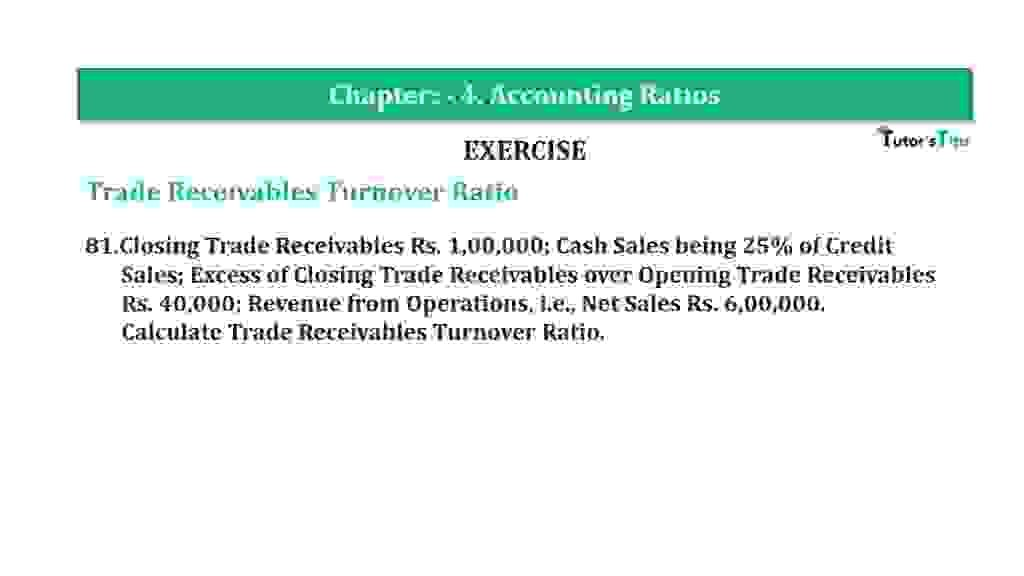# Question 81 Chapter 4 of +2-B – T.S. Grewal 12 ClassQuestion 81 Chapter 4 of +2-B

Interest Coverage Ratio

81.Closing Trade Receivables Rs. 1,00,000; Cash Sales being 25% of Credit
Sales; Excess of Closing Trade Receivables over Opening Trade Receivables Rs. 40,000; Revenue from Operations, i.e., Net Sales Rs. 6,00,000.
Calculate the Trade Receivables Turnover Ratio.

### The solution of Question 81 Chapter 4 of +2-B: –

 Credit Sales = X

 Cash Sales = X x 25 = 25x 100 100

Total Sales = Cash Sales + Credit Sales

 Rs. 6,00,000 = X x 25x + X 100
 Rs. 6,00,000 x 100 = 25x X 100 Rs. 6,00,00,000 = 125x

 X = Rs. 6,00,00,000 125 Credit Sales = Rs. 4,80,000

 Closing Trade Receivables = Opening Trade Receivables + 40,000 Rs. 1,00,000 = Opening Trade Receivables + 40,000 = Rs. 1,00,000 – Rs.40,000 Opening Trade Receivables = Rs. 60,000

 Average Trade Receivable = Opening Trade Receivable + Closing Trade Receivable 2
 Average Trade Receivable = Rs. 1,00,000 + Rs. 60,000 2 = Rs. 80,000

 Inventory Turnover Ratio = Credit Sales Average Trade Receivable
 Inventory Turnover Ratio = Rs. 4,80,000 Rs. 80,000 = 6 Times

Balance Sheet: Meaning, Format & Examples

Thanks, Please Like and share with your friends

Comment if you have any question.

Also, Check out the solved question of previous Chapters: –

### T.S. Grewal’s Double Entry Book Keeping (Vol. II: Accounting for Companies)T.S. Grewal’s Analysis of Financial Statements